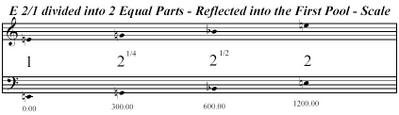Friday, August 05, 2005

Scale of the Day: E Octave Divided into 2 Equal Parts - Reflected Into the First PoolThe E Octave Divided into 2 Equal Parts - Reflected into the First Pool - Scale (as you would find it on any conventionally tuned equal tempered instrument). "Reflected into the First Pool" is a personal convention. The "first pool" is the first interval of a given scale. Reflecting a scale into that interval means mapping the same intervallic proportions into that single interval. This scale is a simple example of this process. An octave divided into two equal parts consists of two "pools" that are 600 cents each. The first "pool" is than divided into two equal parts of 300 cents each creating a "mirror" image of the interval content of the original scale. This is a particularly useful procedure for expanding a scale with severely limited harmonic potential.